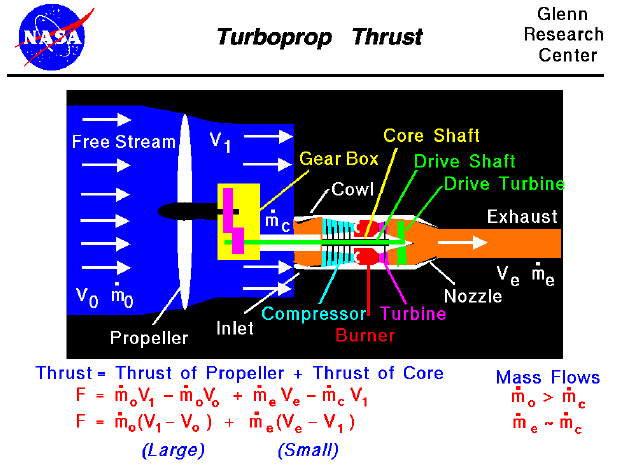To move an airplane through the air, thrust is generated with some kind of propulsion system. Many low speed transport aircraft and small commuter aircraft use turboprop propulsion. On this page we will discuss some of the fundamentals of turboprop engines. The turboprop uses a gas turbine core to turn a propeller. Propellers develop thrust by moving a large mass of air through a small change in velocity. Propellers are very efficient and can use nearly any kind of engine to turn the prop (including humans!). In the turboprop, a gas turbine core is used. How does a turboprop engine work?

There are two main parts to a turboprop propulsion system, the core engine and the propeller. The core is very similar to a basic turbojet having a compressor, burner, and turbine. However, at the exit of the main turbine the hot exhaust gas is passed through an additional turbine, shown in green on the schematic, before entering the nozzle. Unlike a basic turbojet, most of the energy of the exhaust is used to turn this additional turbine. The turbine is attached to an additional drive shaft which passes through the core shaft and is connected to a gear box. The gear box is then connected to a propeller that produces most of the thrust. The exhaust velocity of the core is low and contributes little thrust because most of the energy of the core exhaust has gone into turning the drive shaft.

Turning to the math at the bottom of the slide, the thrust of a turboprop is the sum of the thrust of the propeller plus the thrust of the core. We can use our basic thrust equation on the propeller and core to obtain the thrust equation for the turboprop. We denote the free stream conditions by the subscript "0", the conditions at the exit of the propeller by "1", the exit of the core by "e", and the entrance to the core by "c". The mass flow rate is given by "m dot" and the velocity of the flows by "V". The basic thrust equation then becomes:

F = (m dot)0 * V1 - (m dot)0 * V0 + (m dot)e * Ve - (m dot)c * V1

As we have noted above, the mass flow rate through the propeller (m dot)0 is much greater than the mass flow through the core engine (m dot)c. And we have also noted that the exhaust velocity of the core (Ve) is low and almost equal to the velocity into the core (V1). The mass flow rate exiting the core (m dot)e is almost equal to the mass into the core (m dot)c. (Mathematicians use the symbol "~" for "almost equals.") Collecting this information, we can simplify our basic thrust equation:

F = (m dot)0 * (V1 -V0) + (m dot)e * (Ve - V1)

The first term of this equation is large compared to the second term. Comparing with the pure propeller theory, the thrust is equal to the mass flow through the propeller times the velocity change across the propeller plus a smaller amount of thrust from the core engine.

Because propellers become less efficient as the speed of the aircraft increases, turboprops are used only for low speed aircraft like cargo planes. High speed transports usually use high bypass turbofans because of the high fuel efficiency and high speed capability of turbofans. A variation of the turboprop engine is the turboshaft engine. In a turboshaft engine, the gear box is not connected to a propeller but to some other drive device. Turboshaft engines are used in many helicopters, as well as tanks, boats, and even race cars in the late 1960's.

Guided Tours